# How to Solve Matrices

How to Solve Matrices

In this mini-lesson, we will explore the world of matrices by finding the answers to questions like how to solve systems of equations with matrices, how to solve matrices, and matrix operations with examples and formulas while discovering interesting facts around them.

The word "matrix" was termed by a 19th century English mathematician, James Sylvester.

His friend, Authur Cayley, developed the algebraic aspect of it in the 1850s.

Look at the simulation given below.

Try selecting any of the basic operations listed and see how matrices are solved using them.

## Lesson Plan

 1 How to Solve Matrices? 2 Tips and Tricks 3 Important Notes on How to Solve Matrices 3 Solved Examples on How to Solve Matrices 4 Interactive Questions on How to Solve Matrices

## How to Solve Matrices?

Matrix (plural matrices) is a rectangular array of numbers, symbols, or expressions arranged in $$m$$ rows and $$n$$ columns.

Hence, the order of a matrix is given as $$m \times n$$.

A general matrix may look like:

$\begin{bmatrix} a_{11}&a_{12}&\cdots &a_{1n} \\ a_{21}&a_{22}&\cdots &a_{2n} \\ \vdots & \vdots & \ddots & \vdots\\ a_{m1}&a_{m2}&\cdots &a_{mn} \end{bmatrix}$

For example,

$$\begin{bmatrix} 1 & 2 & 3\\ 4 & 5 & 6\\ \end{bmatrix}$$

Each term in the matrix can be in general written as $$a_{ij}$$, where "$$i$$" is the row and "$$j$$" is the column.

We can solve matrices by performing operations on them like addition, subtraction, multiplication, and so on.

Let's learn about them in detail.

## How to Solve System of Equations Using Matrices?

We have two matrices $$A$$ and $$B$$ where $$A$$ is known as the coefficient matrix and $$B$$ is known as the constant matrix.

There is a third matrix $$X$$ containing all the variables of the equations; this matrix is known as a variable matrix.

Matrix $$A$$ is of the order $$m \times n$$, while $$B$$ is the column matrix of the order $$m \times 1$$.

The product of matrix $$A$$ and matrix $$X$$ results in matrix $$B$$; hence, $$X$$ is a column matrix as well of the order $$n \times 1$$.

The matrices are arranged as:

$$A \cdot X = B$$

Let's understand how to solve a system of equations using matrices with the help of an example.

We have a set of two equations as given below.

The equations are:

$$x + y = 8$$

$$2x + 3y = 10$$

• Arrange all the coefficients, variables, and constants in the matrix in such a way that whenever we find the product of the matrices, the result obtained must result in the equation.

The matrix $$A$$ is:

$$A = \begin{bmatrix} 1 & 1\\ 2 & 3\\ \end{bmatrix}$$

The matrix $$X$$ is:

$$X = \begin{bmatrix} x\\ y\\ \end{bmatrix}$$

The matrix $$B$$ is:

$$B = \begin{bmatrix} 8\\ 10\\ \end{bmatrix}$$

To solve the equations, we need to find matrix $$X$$.

It can be found by multiplying the inverse of matrix $$A$$ with $$B$$, which is given as $$X = (A^{-1})B$$.

To find the inverse of $$A$$, we will need the determinant and adjoint of matrix $$A$$.

To find the determinant of matrix $$A$$, we will follow the below steps:

$$|A| = \begin{vmatrix} 1 & 1\\ 2 & 3\\ \end{vmatrix}$$

Hence, $$|A| = 3 - 2 = 1$$

$$\because$$ $$|A| \neq 0$$, it is possible to find the inverse of matrix $$A$$.

The adjoint of matrix $$A$$ is found by finding each element in it.

Each element in the cofactor matrix is called a minor and found by taking the determinant of the elements leaving the row and column for which the number is to found. The matrix obtained is a cofactor matrix. To find the adjoint of matrix, we take the transpose of the cofactor matrix.

Hence, the adjoint of matrix is given as

$$Adj A = C^T$$

$$C = \begin{bmatrix} C_{11} & C_{12}\\ C_{21} & C_{22}\\ \end{bmatrix}$$

Each term in a cofactor matrix is obtained by $$C_{ij} = (-1)^{i+j} \times M_{ij}$$, where $$i$$ indicates the row, $$j$$ the column and $$M_{ij}$$ being the minor of the matrix.

In this case, the adjoint of the matrix $$A$$ is

$$Adj A = \begin{bmatrix} 3 & -1\\ -2 & 1\\ \end{bmatrix}$$

To find the inverse of $$A$$, we divide the adjoint of $$A$$ by determinant of $$A$$.

Hence, $$A^{-1} = \dfrac{Adj A}{|A|}$$

Applying the same to our example we get,

$$A^{-1} = \begin{bmatrix} 3 & -1\\ -2 & 1\\ \end{bmatrix}$$

Now to find the matrix $$X$$, we'll multiply $$A^{-1}$$ and $$B$$. We get,

$\begin{bmatrix} 3 & -1 \\ -2 & 1 \end{bmatrix} % \begin{bmatrix} 8 \\ 10 \end{bmatrix} \ \begin{bmatrix} 14 \\ -6 \end{bmatrix}$

Hence, the value of matrix $$X$$ is,

$$X = \begin{bmatrix} 14\\ -6\\ \end{bmatrix}$$

 $$\therefore$$ The solution of the equation is $$x = 14$$ and $$y = -6$$Tips and Tricks

• To find the inverse of any ($$2\times2$$), we can use $$A^{-1} = \frac{1}{|A|} \begin{bmatrix} d & -b\\ -c & a\\ \end{bmatrix}$$

Try using this simulation below to observe how equations are solved using matrices.

## What Are Matrix Operations?

The operations that can be performed on matrices are known as matrix operations.

Let's say we have two matrices $$A$$ and $$B$$,

$$A = \begin{bmatrix} a & b\\ c & d\\ \end{bmatrix}$$

$$B = \begin{bmatrix} e & f\\ g & h\\ \end{bmatrix}$$

1. Addition of Matrices

We add the matrices by adding the numbers, symbols, or expressions in the same position.

They are added in the following way: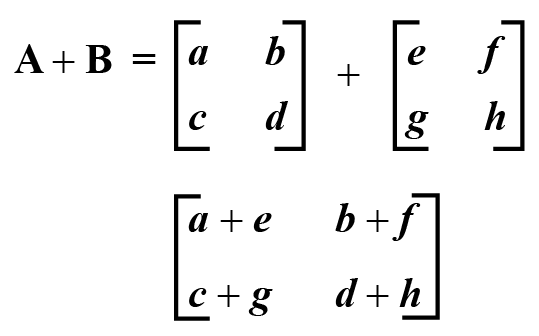1. Subtraction of Matrices

We subtract the matrices by subtracting the numbers, symbols, or expressions in the same position.

They are subtracted in the following way: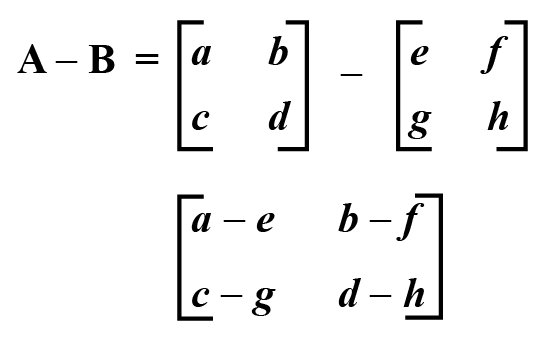1. Multiplying a Matrix by a Constant

On multiplying a constant to a matrix, it gets multiplied in the entire matrix.

It is found as: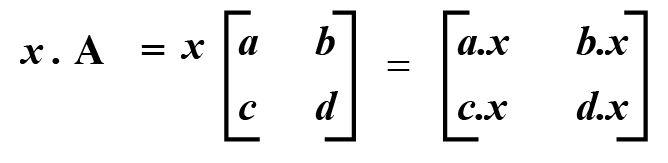1. Negative of a Matrix

The negative of a matrix is found by negating the elements of the matrix.

The negative of a matrix is found in the following way: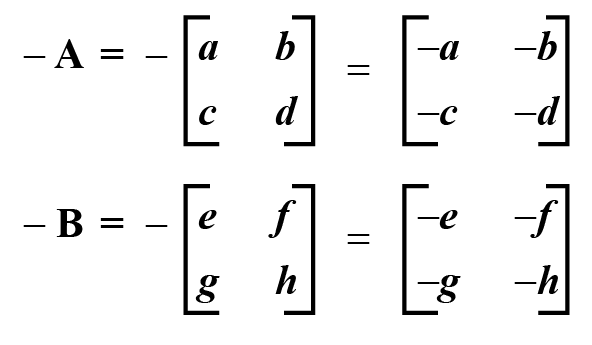1. Multiplying a Matrix by a Matrix

We can multiply a matrix by itself in the following way,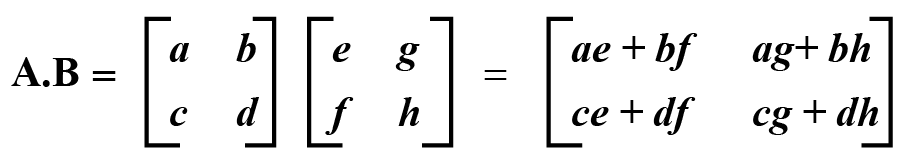1. Transposing a Matrix

The transpose of a matrix can be found in the following way,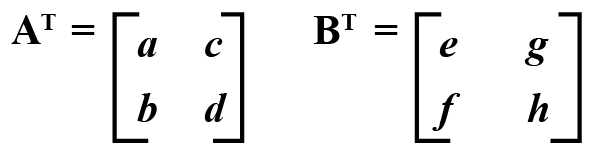Take a look at this simulation below, select your desired operation, and see the output obtained:

### Examples

Example 1: For $$A$$ and $$B$$, find the output of the matrix operations given below.

$$A = \begin{bmatrix} 1 & 2\\ 3 & 4\\ \end{bmatrix}$$

$$B = \begin{bmatrix} 5 & 7\\ 9 & 6\\ \end{bmatrix}$$

1. $$A+B$$
2. $$B-A$$
3. Product of $$A$$ and $$B$$

Solution:

1. Let us say, $$A+B = C$$.

The addition of both the matrices is given as,

$C=\begin{bmatrix} 1 & 2 \\ 3 & 4 \end{bmatrix} + \begin{bmatrix} 5 & 7 \\ 9 & 6 \end{bmatrix} \ \begin{bmatrix} 6 & 9 \\ 12 & 10 \end{bmatrix}$

Hence,

$C = \begin{bmatrix} 6 & 9 \\ 12 & 10 \end{bmatrix}$

 $C = \begin{bmatrix} 6 & 9 \\ 12 & 10 \end{bmatrix}$
1. Let us say, $$D = B-A$$

The subtraction of both the matrices is given as,

$D=\begin{bmatrix} 5 & 7 \\ 9 & 6 \end{bmatrix} - \begin{bmatrix} 1 & 2 \\ 3 & 4 \end{bmatrix} \ \begin{bmatrix} 4 & 5 \\ 6 & 2 \end{bmatrix}$

Hence,

$D = \begin{bmatrix} 4 & 5 \\ 6 & 2 \end{bmatrix}$

 $D = \begin{bmatrix} 4 & 5 \\ 6 & 2 \end{bmatrix}$
1. Let us say, product of $$A$$ and $$B$$ gives $$F$$,

The product of matrices $$A$$ and $$B$$ is,

$F=\begin{bmatrix} 1 & 2 \\ 3 & 4 \end{bmatrix} % \begin{bmatrix} 5 & 7 \\ 9 & 6 \end{bmatrix} \ \begin{bmatrix} 23 & 19 \\ 51 & 45 \end{bmatrix}$

Hence,

$F = \begin{bmatrix} 23 & 19 \\ 51 & 45 \end{bmatrix}$

 $F = \begin{bmatrix} 23 & 19 \\ 51 & 45 \end{bmatrix}$

Example 2: Find:

1. Negative of the given matrix
2. Output when matrix is multiplied by 3
3. Transpose of the given matrix

$A = \begin{bmatrix} -1 & 5 \\ 4 & -2 \end{bmatrix}$

Solution:

1. The negative of the matrix $$A$$ is given as,

$A = -\begin{bmatrix} -1 & 5 \\ 4 & -2 \end{bmatrix}$

The negative of the matrix $$A$$ is,

$A = \begin{bmatrix} 1 & -5 \\ -4 & 2 \end{bmatrix}$

 $A = \begin{bmatrix} 1 & -5 \\ -4 & 2 \end{bmatrix}$
1. The output when matrix is multiplied by 3 is given as,

$B = 3\begin{bmatrix} -1 & 5 \\ 4 & -2 \end{bmatrix}$

The value of the matrix $$A$$ when it is multiplied by 3 is,

$A = \begin{bmatrix} -3 & 15 \\ 12 & -6 \end{bmatrix}$

 $A = \begin{bmatrix} -3 & 15 \\ 12 & -6 \end{bmatrix}$
1. The transpose of given matrix is,

$A^T = \begin{bmatrix} -1 & 5 \\ 4 & -2 \end{bmatrix}^T$

The transpose of $$A$$ is,

$A^T = \begin{bmatrix} -1 & 4 \\ 5 & -2 \end{bmatrix}$

 $A^T = \begin{bmatrix} -1 & 4 \\ 5 & -2 \end{bmatrix}$
More Important Topics
Numbers
Algebra
Geometry
Measurement
Money
Data
Trigonometry
Calculus